## ›  Demo-MAT1: MATLAB-Tutorial

MATLAB is a commercial computing environment developed by MathWorks, targeted specifically at numerical computations and simulations, that uses a proprietary language, also called MATLAB. MATLAB offers functionality for creating and manipulating arrays and matrices, for plotting functions and data and for implementing mathematical algorithms that solve physical and engineering problems. This tutorial gives a brief introduction to the MATLAB language using as environment MATLAB 2019. Basic MATLAB syntax (variables, operators, input, output, arrays, matrices, functions, plotting) is illustrated using small examples that are saved as MATLAB scripts, for example test_hello.m, test_output.m. Most of the examples will also run in the open source software Octave.

###Motivation

A computing environment like MATLAB or Octave offers a broad support for solving mathematical problems analytically as well as numerically. MATLAB programs hide implementation details and much of the underlying complexity. Engineers can set up models and generate solutions even without understanding mathematical model or numerical method very well initially, so they can just focus on their physical problem. Since powerful solvers are available, the focus is shifted from solving a problem to modeling it correctly.

### Why MATLAB?

MATLAB has powerful support for solving numerical problems that model common engineering problems. For example, the Ordinary Differential Equation solvers (ode45, ode23) in MATLAB solve time-dependent problems with a variety of properties. The PDE (Partial Differential Equation) Toolbox provides functions for solving partial differential equations that model structural mechanics and heat transfer problems using finite element analysis. The functions are well-documented and there are many examples available.

### Why Octave?

Octave is a computing environment and scientific programming language very similar with MATLAB and the open source pendant of MATLAB. While the environment is not as sleek as MATLAB, it is free, has the same basic capabilities and is suited for learning MATLAB language basics.

###Overview

The tutorial is structured in sections, that explain the basic MATLAB syntax:

## MATLAB Environment

In order to run MATLAB scripts, a running MATLAB installation is required. You can download a test version from the Mathworks website.

MATLAB's Home-Tab is organized in four windows, that are used for developing and running scripts.

• Folder view: displays the folders that contain m-files. Here you create new folders and new scripts.
• Workspace: displays the variables and functions of the running scripts.
• Editor: displays the contents of the script files. The actual programming is done in the editor.
• Command Window: displays commands and the output of statements.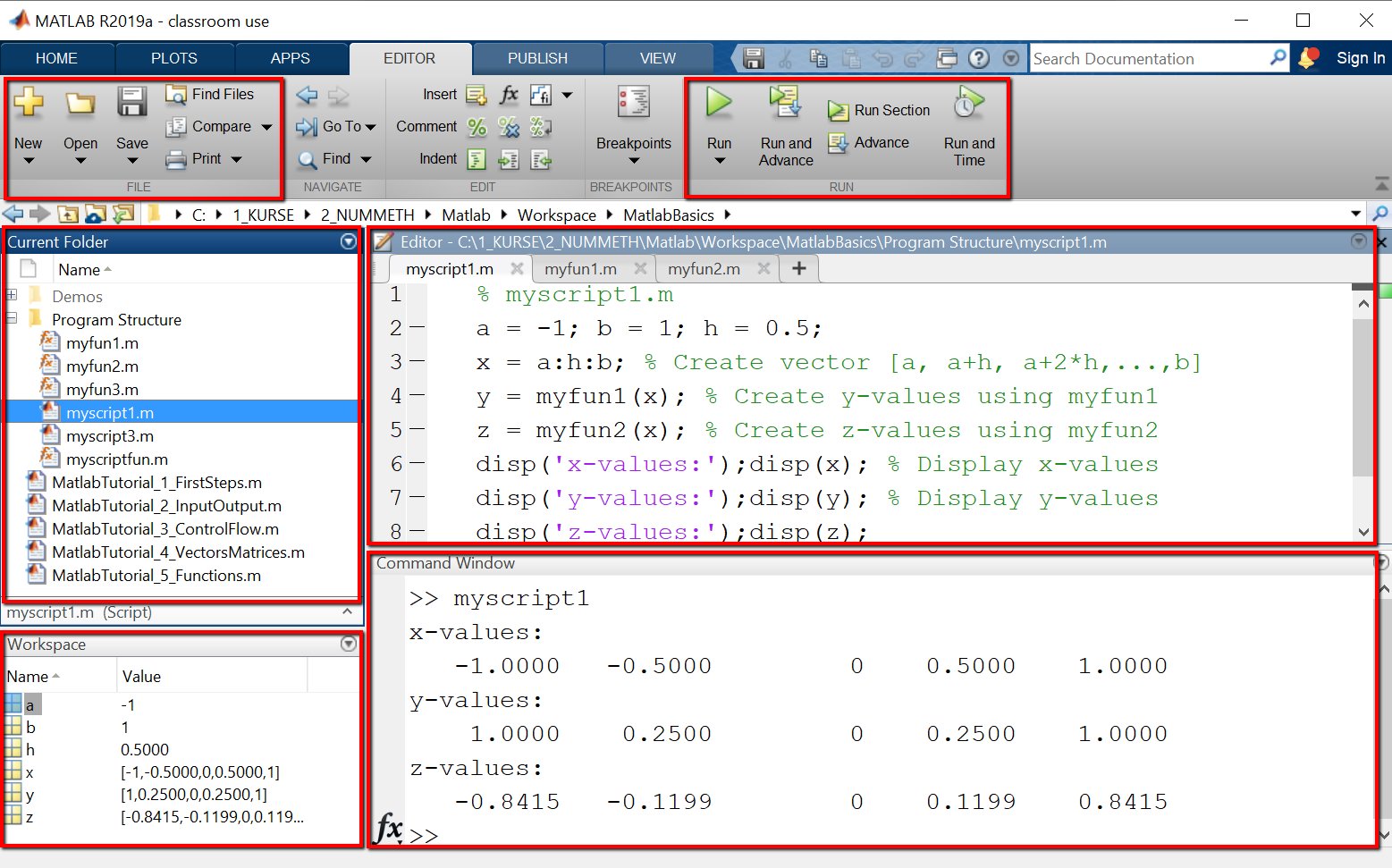A MATLAB program can be created in different ways:

• Interactive mode:
MATLAB can be used in interactive mode, by entering commands directly in the command window. Output is also shown in the command window. Interactive mode is useful for tutorials and for testing small program parts.
• MATLAB Script:
Larger MATLAB programs are collections of MATLAB scripts stored in a common folder. The folder can be anywhere on your computer but must be added to the MATLAB path by using HOME > Set Path Menu.
• MATLAB LiveScript:
MATLAB LiveScripts are a special type of scripts with extended documentation features.

A MATLAB script is a text file with the file type *.m, that contains the statements of the program. MATLAB script names may contain only letters, numbers and subscript. Valid names are for example myscript1.m, myscript2.m, myfun1.m, myfun2.m.
MATLAB scripts are created using the commands in the EDITOR > FILE menu (New, Open, Save etc.) and are executed using the commands in the EDITOR > RUN-Menu (Run, Run and Advance, Run Section).

## First Lines in MATLAB

MATLAB statements can be variable declarations/assignments, operations with variables, input- or output-instructions, control flow statements or function calls, that perform specific tasks (plots, mathematical computations etc.). MATLAB statements can be terminated with a semicolon or not. If terminated with a semicolon, a statement will not generated any output in the command window.
Before starting to explore the MATLAB language, it is useful to learn some commands that are needed frequently to keep a clean and organized workspace and command window.

• clear -- Clears workspace variables. You can delete selected variables or all variables. Useful for example when you run multiple scripts that use the same variable names.
• clc -- Clears command window (instructions and output). Useful when you want to start over with a clean slate.
• format compact -- Compactifies the command window output.
• help -- If you type help folowed by the name of a command or function,

Example: Find out what the clc command does.

When typing "help clc" in the command window, the documentation for the clc command is shown as below.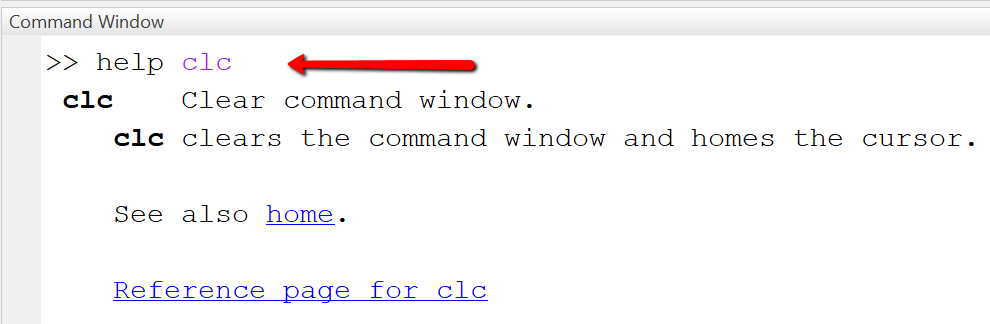### Hello World-Programm in MATLAB

A Hello-World-program is the smallest runnable program in a programming language and outputs the Text "Hello World" to default output (here: the command window). We do it first in interactive mode and then in a script.

Interactive mode
In interactive mode, we use only the command window. Statements are entered next to the the command prompt (indicated by >>) and executed by pressing ENTER.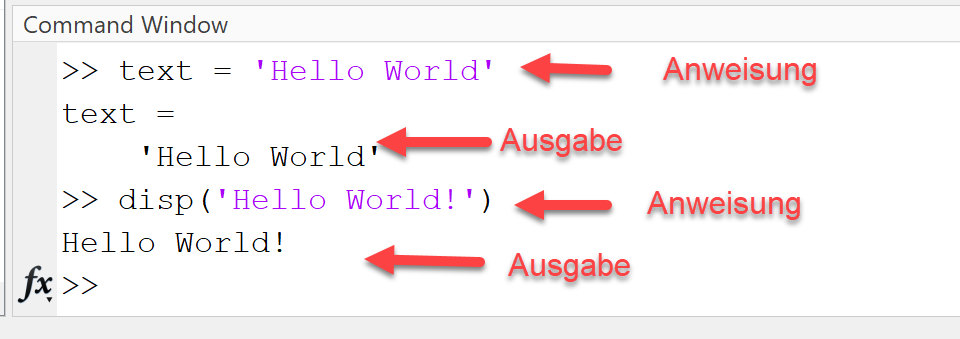We type the statement text='Hello World' in the command window and then press ENTER. As shown in the picture below, the variable (here: text) and its content (here: Hello World) are shown as output in the command window. Next, we type the statement disp('Hello World') and press ENTER. Again, the string is displayed, but this time without the name of the variable.

Script mode
In script mode, we first create a new script with the name "test_hello.m", then type the MATLAB statements below in the script file and save it, and finally execute the script by clicking the "RUN"-Button.

The MATLAB platform after creating, editing, saving and running the script looks as shown below.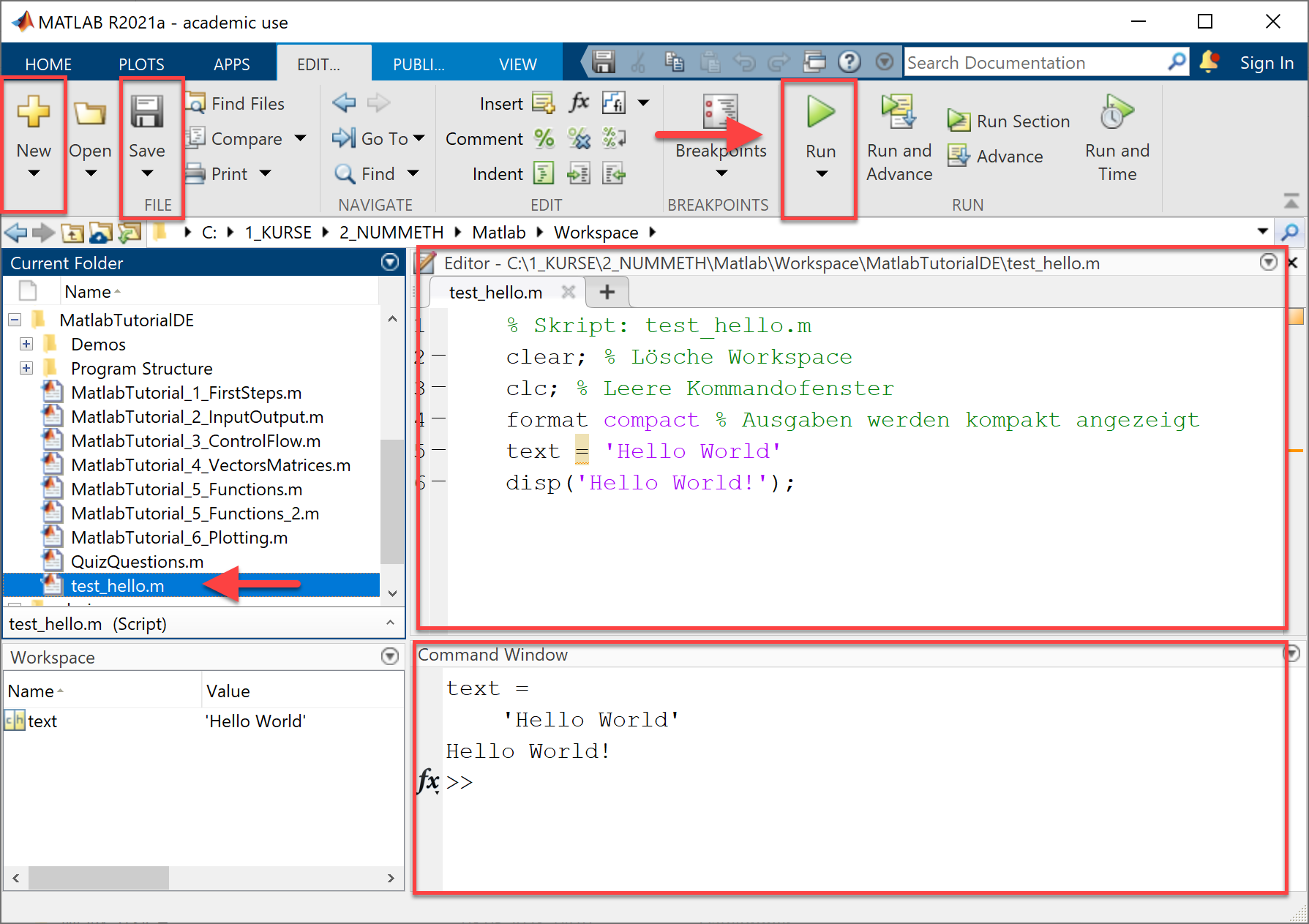The code starts in line 1 with a comment (indicated by "%"-symbol), that states the name of the script file. Note that the following lines are also commented.
In lines 2 and 3 we insert code for resetting / preparing workspace and command window.
In line 4 we create a new variable "text" and assign it the value 'Hello world'.
In line 5 we print the string 'Hello World' using disp().

```` % Script: test_hello.m`
` clear;clc; % Clear workspace and command window`
` format compact % Compactify command window output`
` text = 'Hello World'`
` disp('Hello World!');`
```

Comments in MATLAB start with a "%"-symbol followed by text. Comments are used for documentation purpose, they are not executed by the system. Two percent-symbols "%%" mark a section. Sections can be executed separately using the RUN SECTION-Button.

```` %% Section 1`
` % Comment 1: This is the first comment for section 1.`
` text = 'Let''s start simple' % Here we assign a value to the variable named text`
` %% Section 2`
` % Comment 2: This is the first comment for section 2.`
` a = 10 % Here we assign a value to variable a`
` b = 20 % Here we assign a value to variable b`
```

### Variables and data types

Variables are named memory spaces that store the data of your program. MATLAB variables are declared by simply assigning a value to them. The data type (number, string, array etc.) is assigned automatically by MATLAB. For example, all numbers (integer, floating points) are stored internally as double precision floating point numbers. Knowing the data type is important, since the data type determines the set of operations that you can perform with your variables.

Example: Using variables
The example shows how to declare variables and perform operations on them. Note that the +-symbol denotes different operations: in line 3, the addition of numbers, in line 5, the concatenation of strings.

When running the script test_variables.m, output in command window is as shown.

Code Output
```` % test_variables.m`
` a = 10; b = 20.5;`
` sum = a + b`
` s1 = "apples";s2 = "pears";`
` text1 = s1 + "+" + s2`
` text2 = replace(text1, '+',' or ')`
```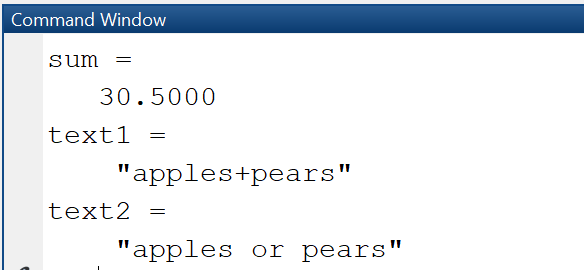Code explanation:

• Line 2: Declare two variables a, b by assigning values to them. Since the declarations are terminated with semicolon, output of the variables to the command window is suppressed.
• Line 3: Add the two variables (actually, their values) and store the result in the variable sum.
• Line 4: Declare two string variables s1, s2.
• Line 5: Concatenate the strings to form a new string.
• Line 6: Replace the "+"-character with the string ' or '.

### Data types

MATLAB supports different data types, that are represented by classes:

• Numerical data types: int, single, double
• Boolean data types: logical
• String data types: string, char

In order to find out the exact data type and size of your variables, use the commands whos, class. With whos, you can find out size and type of workspace objects. With class, you can find out the data type of a variable.

Script Output
```` a = 10;`
` b = 20.5;`
` whos a`
` whos b`
```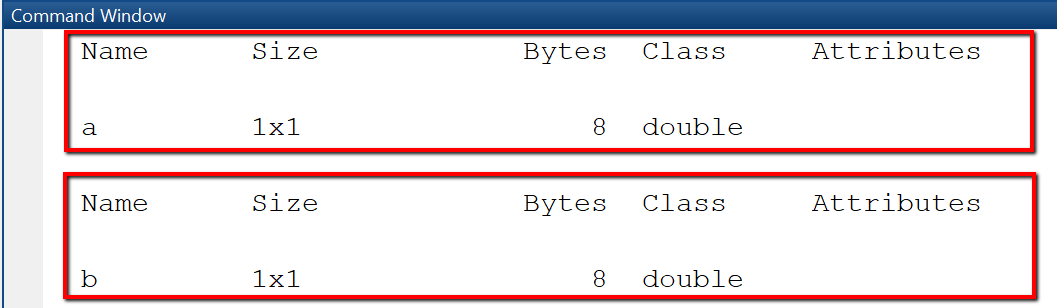### String variables

String variables in MATLAB can be either of class "string" or of class "character array". When you create a string using single quotes, it is stored as character array. When you create a string using double quotes, it is stored as a string. A character array is a sequence of characters, just as a numeric array is a sequence of numbers. String arrays provide a set of functions for working with text as data.

Script Output
```` text1 = "Hello"`
` whos text1`
` text2 = 'from Kaiserslautern'`
` whos text2`
```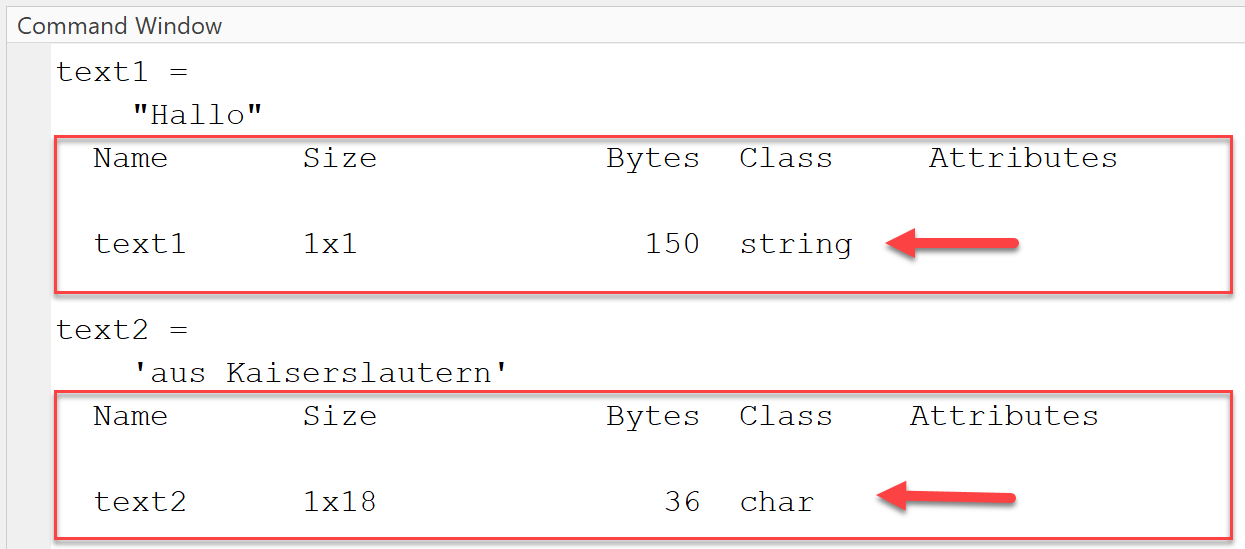## Output and Input

Data stored in variables can be viewed in the workspace or displayed in the command window.

### Output to command window

There are three ways to output variable values to command window.

• Type the name of a variable in a new line, with no terminating semicolon. In an assignment statement, omit semicolon at the end of a statement. The content of the variable then is displayed in the command window.
• Use disp()-function, This functions takes as argument a variable / vector / matrix and displays it, omitting the name of the variable.
• Use fprintf()-function. This function builds a formatting string by using placeholders for the variables to be inserted. In our example, in the place indicated by the first "%d" the value of variable a, which must be an interger number, will be inserted.

Example: Output to command window
In this example we calculate the sum of two variables and generate output in the command window.

Script Output
```` % test_output.m `
` a = 10; b = 20; sum = a + b;`
` % (1) No semicolon`
` disp('(1) Output by typing variable name')`
` sum % output: sum = 30`
` % (2) Use disp`
` disp('(2) Output using disp()')`
` disp([a b sum]) % output: 10 20 30`
` % (3) Use fprintf for formatted output`
` disp('(3) Output using fprintf')`
` % output: a = 10, b = 20, Sum = 30`
` fprintf('a = %d, b = %d, Sum = %d\n', a, b, sum)`
```

When running the script test_output.m, output looks as displayed.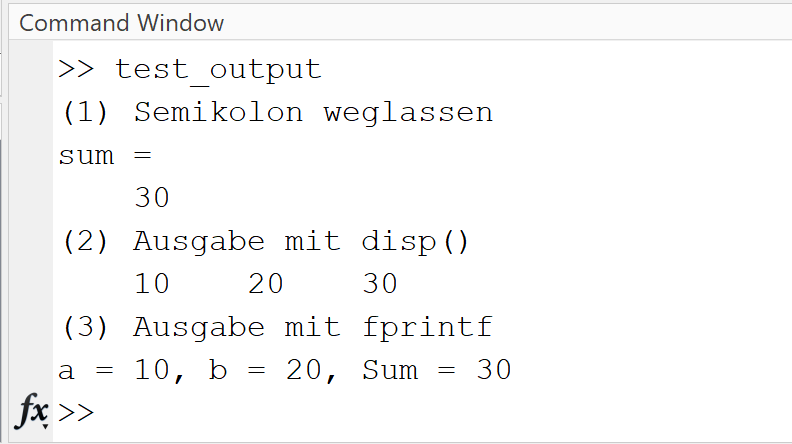### Input from command window

When presenting a MATLAB script to non-technical users, it may be helpful to let them enter configuration parameters as input from the command window or an input dialog. Input entered in the command window can be stored in variables using the input(prompt)-function.

Script Output
```` % Display a prompting text`
` prompt = 'Enter a: ';`
` % Read input`
` a = input(prompt);`
` prompt = 'Enter b: ';`
` b = input(prompt);`
` sum = a + b;`
` fprintf("Sum = %.2f\n", sum);`
```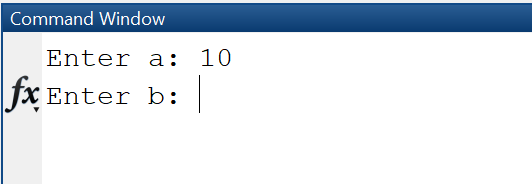### Input dialog

An input dialog as shown below is created using the MATLAB-function inputdlg. We pass four arguments to the function:

• prompt -- the labels of the input fields
• dlgtitle -- the title of the dialog
• windowsize -- the size of the window
• definput -- specifies the default value for each edit field. The definput argument must contain the same number of elements as prompt.

The function returns an array of strings "answer", that contains the values entered in the edit fields.

Script Output
```` prompt = {'Enter a:', 'Enter b:'};`
` dlgtitle = 'Input';`
` windowsize = [1 50];`
` definput = {'10','20'};`
` answer = inputdlg(prompt, dlgtitle, windowsize, definput)`
` a = str2num(answer{1})`
` b = str2num(answer{2})`
```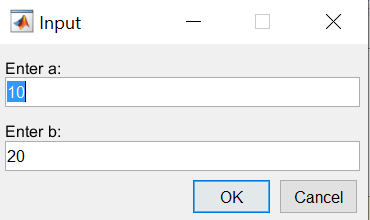## Control flow

Control flow statements are used to determine which block of code to execute at run time (conditional statements, if-else) or to execute a block of code repeatedly (while-loop, for-loop).

### Conditional statements (if-else)

Conditional statements are used to control which of two or more statement blocks are executed depending on a condition. In MATLAB, as in most programming languages, they are implemented using the if-else-syntax.

Example: Show message according to the size of the number
In this example the user is asked to enter a number smaller that 100 and according to the size of the input a message is displayed. In line 5, the condition "a < 30" is evaluated: If true, the statement in line 5 is executed and the program flow continues in the first line after the if-else-statement.

Script Output
```` % test_ifelse.m`
` prompt = 'Enter number < 100: ';`
` a = input(prompt);`
` disp('Your number:'); disp(a); % display it`
` if a < 30`
`     disp('Small number!')`
` elseif a < 80`
`     disp('Medium number!')`
` else`
`     disp('Large number!')`
` end`
```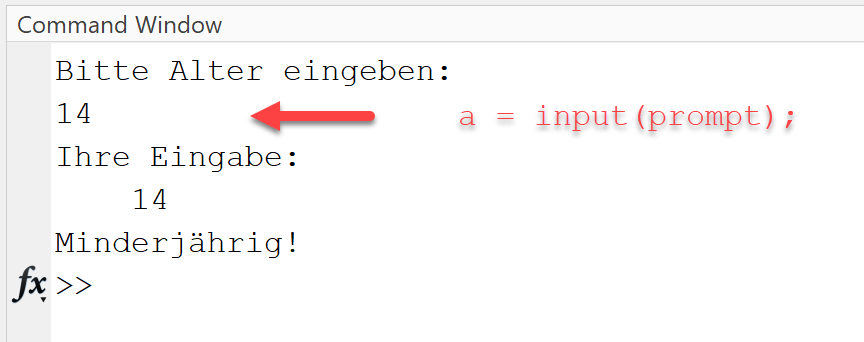### Loops

A While loop repeats the statements in the loop while a condition is evaluated as true.

Example: Calculate sum of first n numbers: sum = 1+2+...+n
In this example, a counter variable i is initialized with 1. In line 3 the loop condition "i <= 5" is checked. If "true", the statements in line 4-6 are executed (print the value of i, add the value of i to the value of sum, increment the value of i) and then the loop condition is checked again and the loop is repeated.

Script Output
```` sum = 0;`
` i = 1; % (1) Initialize counter i`
` while i <= 5 % (2) Check condition`
`     fprintf("%d + \n", i);`
`     sum = sum + i;`
`     i = i+1; % (3) Increment counter   `
` end `
```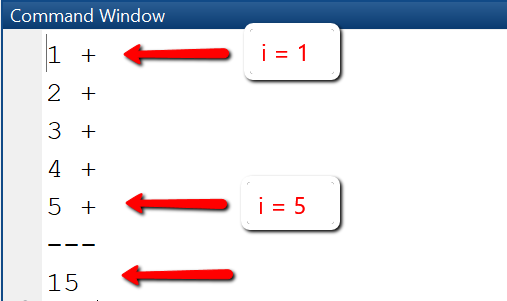A For loop repeats the statements in the loop a specified number of times.

Script Output
```` n = 6; sum = 0;`
` % Start at index 1, use increment 2, end at index n `
` for i=1:2:n `
`     fprintf("%d + \n", i);`
`     sum = sum + i;`
` end`
` fprintf("---\n");`
` fprintf("%d\n", sum);`
```## Vectors

Vectors are one-dimensional arrays that store information. They can be stored in row or column format. For example, x = [1 4 9] will be a row vector, and x = [1; 4; 9] will be a column vector. Vectors are created in different ways: by listing their elements explicitly in square brackets, using functions, or using for-loops.
Elements of a vector are accessed using an index that starts at 1. For example, to access element i in vector x, we write x(i).

Example: Create vector with 5 elements
We first create a row vector with 5 elements, display the second element of the vector, then the elements 2, 3 and 4 and change element 3 to be the sum of the first two elements.

Script Output
```` x = [1 4 9 16 25]`
` disp('Element 2 is: ')`
` disp(x(2))`
` disp('Elements from 2 to 4 are:')`
` disp(x(2:4)) `
` % Change element 3 to be sum of element 1 and 2`
` disp('Vector x after changing 3rd element:')`
` x(3) = x(1) + x(2)`
```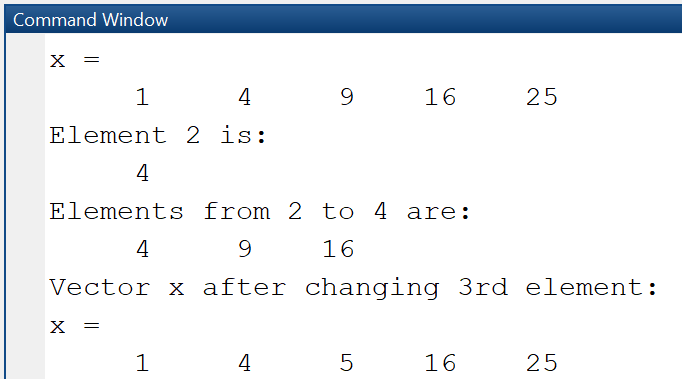Example: Find out size and length of vector
Using the whos command, we can see that the vector x is actually a matrix with 1 row and 5 columns, is of class double and uses 40 bytes of storage.

Script Output
```` x = [1 4 9 16 25]`
` disp('Whos x:')`
` whos x`
` disp('Size of x:')`
` sz = size(x)`
` disp('Length of x:')`
` n = length(x)`
```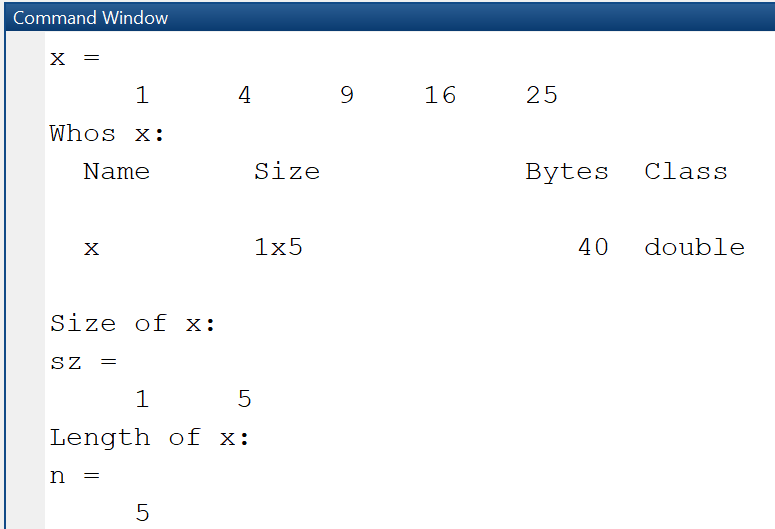### Vector operations

MATLAB supports vector operations of two kinds: mathematical operations according to the rules of linear algebra (transposition, addition, multiplication) and array-type elementwise operations. Elementwise operations are distinguished from vector operations by usage of the dot character (.).
x * y means matrix multiplication, and works only if x is a 1xn row vector and y is a nx1 column vector.
x .* y means elementwise multiplication and works only if x and y are both row (or column) vectors of same size.

Vector and elementwise operations are the same for addition and subtraction, so the operators .+ and .- are not used. Since vectors actually are a special type of matrix, these operations are the same as the described below for matrices.

Script Output
```` x = 0:2:8 % x = 0,2,4,6,8`
` % Add 1 to each element`
` y = x + 1 % y = 1,3,5,7,9`
` % Multiply x and y elementwise`
` z = x .* y % z = 0*1+2*3+...+8*9`
` % Slicing`
` % Extract a slice from 2 to 5`
` xslice = x(2:4) % x1 = 2,4,6`
```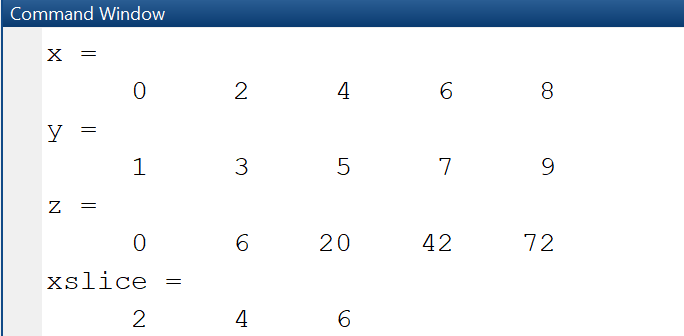## Matrices

Data of MATLAB programs are stored in one- and two-imensional arrays (e.g. vectors and matrices). A good understanding of matrices is important, since variables and vectors are actually a special case of matrices. Matrices are created in different ways: by enumerating their elements explicitly in square brackets, using functions, or using for-loops.

Create matrix using enumeration
When declaring a matrix by explicit enumeration, row elements are separated by a space and columns are separated by semicolon.

Create matrix using functions
Many mathematical problems require to use matrices initialized with specific values, such as the identity matrix. In MATLAB, the declaration of these matrices is done using MATLAB functions such as zeros(), ones() or eye().

Create matrix using for loops
The creation of a matrix using for loops is required when the size and even the element values change dynamically depending on some parameters. In this case, it is recommended to preallocate the maximal required memory using the zeros()-function.

Elements of a matrix are accessed using a row index and a column index that both start at 1. For example, to access element in row i and column j in a matrix A with m rows and n columns, we write A(i, j). Element with row-index 1 and column-index 1 is accessed with A(1, 1), etc.

Example: Create matrix by enumeration
In this example we declare a matrix with two rows and three columns, display it using disp, and find out type and size using whos. The whos-command shows that it is indeed a 2x3 matrix and uses up 48 bytes of memory.

Script Output
```` A = [1 2 3;4 5 6]; % 2 rows, 3 cols`
` disp('Matrix A:')`
` disp(A)`
` whos A`
```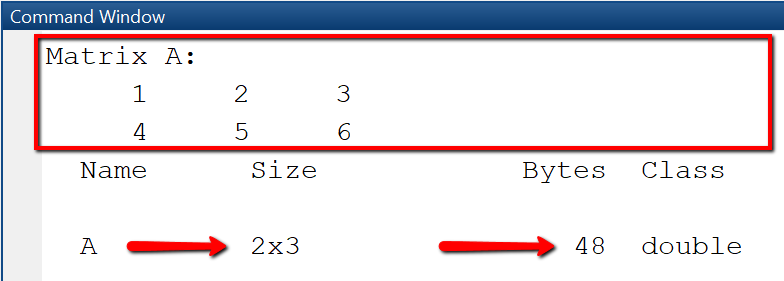Example: Create matrices using MATLAB functions
In this example we create three matrices: A1 is a matrix with m rows and n columns whose elements are initialized with zeros. A2 is a mxn matrix whose elements are all initialized with 1. I_n is the identity matrix with n rows and n columns, with ones on the diagonal and 0 on all other elements.

Script Output
```
` m = 2; n = 3; `
` % mxn matrix with elements initialized to 0`
` A1 = zeros(m, n) `
` % mxn matrix with elements initialized to 1`
` A2 = ones(m, n) `
` % nxn identity matrix with 1 on diagonal`
` I_n = eye(n, n) `
```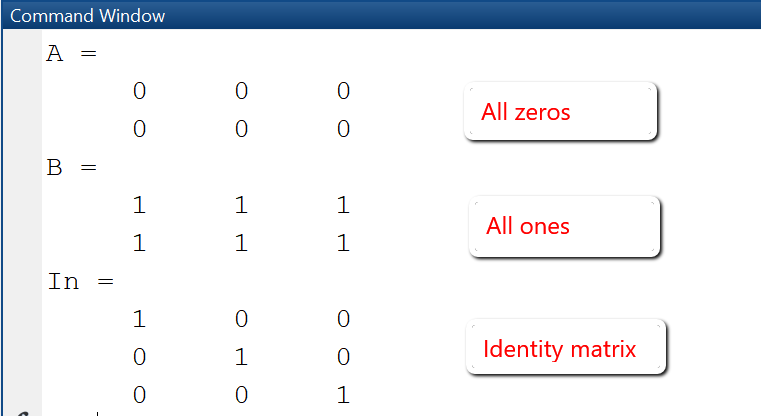Example: Extract elements from a matrix
Accessing elements from a matrix is done by using indexing and slicing. In this example we first extract element with row-index 2 and column-index 3 and store in in a variable el23, then we extract the rows from 1 and 3 and all columns and store the result in a new matrix A1, finally we extract the rows from 2 to 3 and columns from 1 to 2 and store the result in a new matrix A2.

Script Output
```` A = [1 2 3; 4 5 6; 7 8 9]`
` el23 = A(2,3) % el23 = 6: `
` % Extract rows 1 and 3`
` A1 = A([1,3],:) % A1 = [1 2 3; 7 8 9]`
` % Extract rows 2 and 3 and and columns 1 and 2`
` A2 = A(2:3,1:2) % A2 = [4 5; 7 8]`
```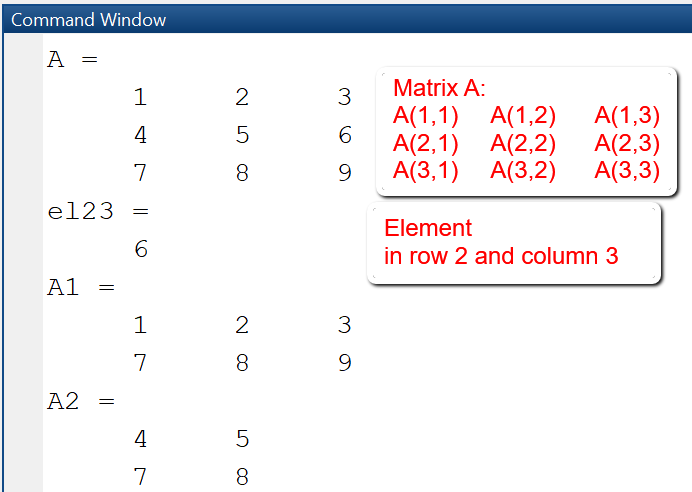### Matrix operations

MATLAB supports matrix operations of two kinds: mathematical operations according to the rules of linear algebra (transposition, addition, multiplication) and array-type elementwise operations. Elementwise operations are distinguished from matrix operations by usage of the dot character (.): A * B means matrix multiplication, A .* B means elementwise multiplication. Since matrix and elementwise operations are the same for addition and subtraction, the operators .+ and .- are not used.

Example: Matrix operations
This example shows frequently used matrix operations: transposition, addition, multiplication and elementwise multiplication. The transposed of a matrix A is obtained by writing A', i.e. the matrix name followed by a quotation mark.

Script Output
```` % Transpose a matrix`
` A = [1 2; 3 4]`
` B = A' % B = [1 3; 2 4]`
` % Matrix addition`
` A = [1 2; 3 4];B = [1 3; 2 4];`
` C = A + B % C = [2 5; 5 8]`
` % Matrix multiplication`
` A = [1 2; 3 4];B = [1 3; 2 4];`
` D = A * B % D = [5 11; 11 25]`
` % Elementwise multiplication`
` A = [1 2; 3 4];B = [1 3; 2 4];`
` E = A .* B % E = [1 6; 6 16]`
```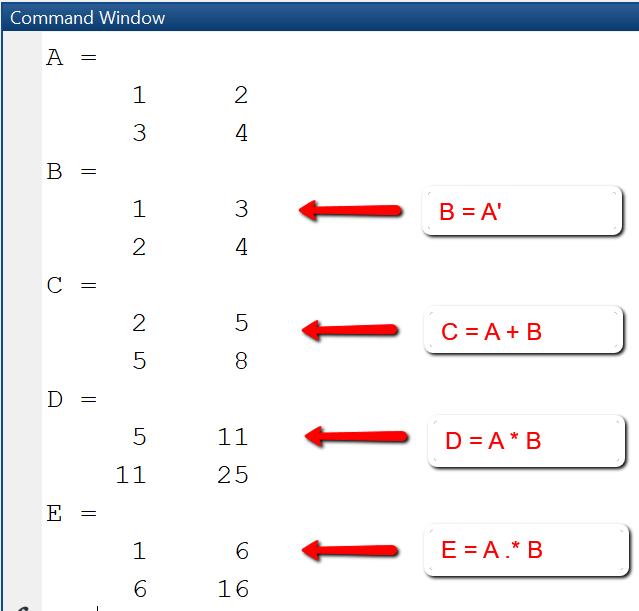## Functions

A function is a reusable piece of code that is executed only when called. It is defined once and can be called multiple times. Functions may have parameters and also may return parameters. The most common application is to use predefined MATLAB-functions such as plot (for plotting) or linspace for creating equidistant vectors, or solvers such as ode45.
User-defined functions can be defined in multiple ways:

• Function is stored in a separate m-file.
• Function is stored inline in the script where it is called.
• Function is defined as an anonymous function.

### (1) Function stored in a separate m-file

In most cases, it is recommended to put the code of a function in its own function script.
A function script is a MATLAB m-file that contains only the definition of a single function and cannot be executed as a "normal" script. Function and function script must have the same name, for example a function with name myfun1 must be stored in a function script with name myfun1.m.
By storing a function in its own script, the function can be used by any other script in the MATLAB path by calling it with specific arguments.

Defining a function
Functions begin with the keyword "function", followed by the statement
outputargs = function_name(inputargs)
and end with the keyword "end". The actual function statements that define the relationship between the input arguments and the output arguments are written in the function body between the first line and the last line.
In order to display the general syntax of a function definition and generate a template for a new function, we use the New > Function-Button in the EDITOR-Tab. This creates a new function script "untitled.m" for the function "untitled" with two generic input arguments and two generic output arguments. Starting with this template, we create our own functions by changing name, arguments and code as needed.

```` function [outputArg1,outputArg2] = untitled(inputArg1,inputArg2)`
` %UNTITLED Summary of this function goes here`
` %   Detailed explanation goes here`
` outputArg1 = inputArg1;`
` outputArg2 = inputArg2;`
` end`
```

Example: Define and call a function myfun1
In this example, we create a function myfun1 that takes as input an array-parameter x and calculates the function values y = x*exp(-x2). The code to the left shows the function definition in the function script. In the test script myscript1.m we first create an equidistant vector x, then calculate the y-values by calling the function myfun1 and finally create a plot of the so created x,y-values.

MATLAB's plot-function is used to create 2D-line plots of data given as (x,y)-values. The plot-function takes as input two vectors that must be of same size, plus a number of formatting options for specifying line type, color, width, title, legend, axis labes etc.

Script Output

Function myfun1.m

```` function y = myfun1(x)`
` % Define the function y = x exp(-x^2)`
` %   Input: x -- a vector`
` %   Output: y -- a vector (same size as x)`
` y = x .* exp(-x.^2);`
` end`
```

Script myscript1.m

```` x = -5:0.1:5; % Create vector x`
` y = myfun1(x); % Calculate y`
` plot (x, y, '+-'); % Plot y vs. x`
` title('y(x) = x * exp(-x^2)');`
` xlabel('x'); ylabel('y');`
```### (2) Function stored inline in main script

Functions that will be used only in one script can be stored inline in that script. In order for this to work, the following syntax rules hold:
- the one script that stores everything (main program and helper functions) must be declared as function script, i.e. start with the keyword "function" followed by an user-defined name.
- the helper functions are defined below the main program
- the functions are not allowed to have a terminating "end"-command.

Example: Define and plot two functions
In this example, we create two functions myfun1 and myfun2 inline in the test script "test_inlinefunction.m". The test script "test_inlinefunction.m" must also start with the keyword "function", however, this function has no input and output parameter and simply indicates that the script will contain inline functions. Both functions myfun1 and myfun2 have as input parameter a vector x, and calculate the y-values for given mathematical functions.

Script Output
```` function test_inlinefunction`
` x = -1:0.1:1; `
` plot(x, myfun1(x), '+-');`
` hold on`
` plot(x, myfun2(x), '+-');`
` legend('myfun1','myfun2');`
` title('Line-plot of two functions');`
` xlabel('x'); ylabel('y');`
` function y = myfun1(x)`
`    y = sin(x) .* exp(-x.^2);`
` function y = myfun2(x)`
`    y = cos(x) .* exp(-x.^2);`
```### (3) Anonymous functions

An anonymous function is a function that is not stored in a script file, instead it is associated with a variable of type function_handle. Informally, it is an inline function that is written in just one line with the help of the @-symbol. Anonymous functions are used for functions whose defining statement can be written in just one line.
General syntax is <function_name> = @ (<param_list>) ( <computation>).

Example: Use anonymous functions
In this example three mathematical functions are defined as anonymous functions.

• Line 1-3: Define function f(x) = sin(2πx) and then calculate the function values at points x = 1 and x = 2.
• Line 4-8: Define polynomial p(x) = 1 + 2x + x2 and then calculate the function values at the equidistant points x = [0, 0.5, 1.0, 1.5, 2.0]. Since in the definition of the polynomial the dot-operator is used, it is allowed to pass a vector as argument to the function.
• Line 8-11: Define a function of two variables u(x,y) = 25xy, where x and y are allowed to be vectors of same size. Then, create two vectors x, y using linspace and calculate the function values z for these vector arguments.
```` % Example 1: Sin-Function`
` f = @(x) sin(2*pi*x);`
` y1 = f(1); y2 = f(2);`
` % Example 2: Polynomial`
` p = @(x) (1 + 2.*x + x.^2);`
` x = 0:0.5:2; % x = [0, 0.5, 1.0, 1.5, 2.0]`
` y = p(x);`
` % Example 3: Function with two variables`
` u = @(x,y)(25.*x.*y);`
` x = linspace(0,1,10); y = linspace(0,2,10);`
` z = u(x, y);`
```

Contributors:
Prof. Dr. Eva Maria Kiss
Tools: MATLAB, Octave